# RBSE Maths Class 9 Chapter 1: Vedic Maths Important Questions and Solutions

Vedic Maths Class 9 RBSE Important questions and solutions are provided here. All these questions have stepwise solutions, which aim at a student’s better understanding. The important questions and solutions available at BYJU’S for Vedic Maths will help the students to clear their doubts and to get full marks for the questions from this chapter, in their final exams.

Vedic Maths Chapter 1 of RBSE Class 9 will help the students to perform the mathematical operations quickly. As the methods involved in this chapter are easy, less time consuming and short too. The results obtained by these Sutra’s based methods can also be verified manually to get more confidence.

## In RBSE Class 9 Maths Chapter 1, we will help you with:

• RBSE Chapter 1: Exercise 1.1 Textbook Important Questions and Solutions
• RBSE Chapter 1: Exercise 1.1 Textbook Important Questions and Solutions
• RBSE Chapter 1: Additional Important Questions and Solutions

### RBSE Maths Chapter 1: Exercise 1.1 Textbook Important Questions and Solutions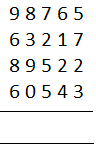Solution:

(i) Column I (from the right side), 5 + 7 = 12

(ii) Mark Ekadhika dot(.) on 1, (digit which is next to 7 in the column II)

(iii) Start again adding with 2

(iv) 2 + 2 = 4, Again 4 + 3 = 7

(v) Write 7 below at the answer’s place

(vi) Add the remaining columns in the same way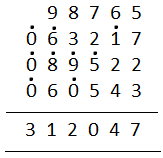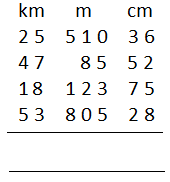Solution:

(i) Column I (from the right side), 6 + 2 = 8

(ii) Continue adding 8 + 5 = 13

(iii) Mark Ekadhika dot(.) on 1, (digit which is next to 7 in the column II)

(iv) Start again adding with 2

(v) 3 + 8 = 1

(vi) Mark Ekadhika dot(.) on 2

(vii) Write 1 below at the answer’s place

(viii) Add the remaining columns in the same way and keep the sum in terms of digits, as per the specified column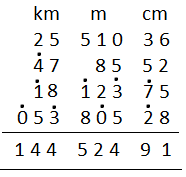Question 3: By vedic method subtract the following: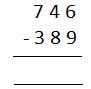Solution:

Steps for subtraction:

(i) Column I, 9 cannot be subtracted from 6. So the nearest larger number is 8. Add 8 + 9 = 17. Write 7 in the answer place.

(ii) Mark Ekadhlkena dot at digit 8

(iii) Similarly, follow the same procedure for remaining columns.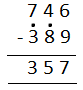Question 4: By vedic method subtract the following: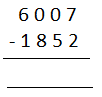Solution:

Steps for subtraction:

(i) Column I, 7 – 2 = 5.

(ii) Write 5 in the answer place.

(iii) Similarly, follow the same procedure for remaining columns.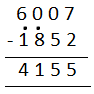Question 5: By Sutra Ekadhikena Purvena multiply the following:

42 × 48

Solution:

(i) Sum of Charaman digits 2 + 8 = 10

(ii) Remainder Nikhilam digits are equal to 4

(iii) Two digits in RHS = 16

42 × 48

= 4 × 5/2 × 8

= 20/16

= 2016

413 × 487

Solution:

(i) Sum of Charaman digits 13 + 87 = 100

(ii) Remainder Nikhilam digits are equal to 4

(iii) Two digits in RHS = 1131

413 × 487

= 4 × 5/ 13 x 87

= 20/1131

201131

Question 7: Multiply the following with the help of Sutra Ekanyunena Purvena.

54 × 99

Solution:

LHS: 54 – 1 = 53

RHS: 99 – 53 = 46

54 × 99 = 54 – 1 / 99 – 53

= 53 / 46

= 5346

Question 8: Multiply the following with the help of Sutra Ekanyunena Purvena.

342 × 99999

Solution:

First make the number of digits for both multiplicand and multiplier equal.

Thus,

00342 x 99999

LHS: 00342 – 1 = 00341

RHS: 99999 – 00341 = 99658

00342 × 99999 = 00342 – 1 / 99999 – 00341

= 0034199658

Question 9: By Vedic method multiply the following.

$$\begin{array}{l}15\frac{5}{7}\times 15\frac{2}{7}\end{array}$$

Solution:

(i) Sum of the fractions = (5/7) + (2/7) = 7/7 = 1

(ii) Remainder Nikhilam digits are equal to 15

$$\begin{array}{l}15\frac{5}{7}\times 15\frac{2}{7}=15 \times 16 / \frac{5}{7}\times \frac{2}{7}\end{array}$$

$$\begin{array}{l}=240 / \frac{10}{49}\\ =240\frac{10}{49}\end{array}$$

Question 10: By Vedic method multiply the following.

9.85 x 9.15

Solution:

(i) Sum of decimal fractions = 0.85 + 0.15 = 1

(ii) Remainder Nikhilam digits are equal to 9

9.85 x 9.15

= 9 x 10 / 0.85 x 0.15

= 90 / 0.1275

= 90.1275

### RBSE Maths Chapter 1: Exercise 1.2 Textbook Important Questions and Solutions

Question 11: Change 878 into Vinculum number.

Solution:

(i) Subtracting the unit digit from 10, 10 – 8 = 2

(ii) Subtract the remaining digits from 9, 9 – 7 = 2, 9 – 8 = 1

(iii) Drae vinculum bar on 1 and 2

(iv) Mark Ekadhikena dot on 0, which is prior to 1 bar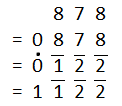Question 12: Change 9687 into Vinculum number.

Solution:

(i) Subtracting the unit digit from 10, 10 – 7 = 3

(ii) Subtract the remaining digits from 9, 9 – 8 = 1, 9 – 6 = 3, 9 – 9 = 0

(iii) Draw vinculum bar on 1 and 3

(iv) Mark Ekadhikena dot on 0, which is prior to 0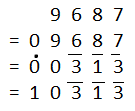Question 13: Change into an ordinary number.

$$\begin{array}{l}3\bar{2}1\end{array}$$

Solution:

(i) Subtract the positive value of Charmam digit (unit digit) from 10

(ii) Write the remaining digits as it is

(iii) Place an Ekanyunena dot beneath the non Vinculum digit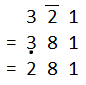Question 14: Change into an ordinary number.

$$\begin{array}{l}450\bar{4}\bar{9}\end{array}$$

Solution:

(i) Subtract the positive value of Charmam digit (digit under bar) from 10

(ii) Subtract the positive values of remaining Nikhilam digits (which are under bar) from 9

(iii) Write the remaining digits as it is

(iv) Place an Ekanyunena dot beneath the non Vinculum digit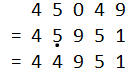Question 15: Multiply the following by Sutra Nikhilam.

102 × 107

Solution:

Steps:

(i) Base = 100

(ii) Deviations from base 100 are +2 and +7

(iii) Two digits should be in RHS, keep 14 in RHS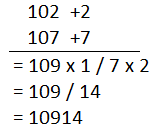Question 16: Multiply the following by Sutra Nikhilam.

72 × 73

Solution:

Steps:

(i) Base = 10, sub-base = 10 × 7 = 70

(ii) Deviations from base 70 are +2 and +3

(iii) One digit should be in RHS, keep 6 in RHS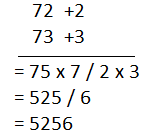Question 17: Multiply the following by Sutra Nikhilam.

99 × 101 × 103

Solution:

99 × 101 × 103

Considering base for the numbers as 100

 Number Deviation 99 -1 101 +1 103 +3

Taking 99 and adding deviations of other two numbers, sum of product of two deviations and product of three deviations.

= 99 + 1 + 3 / 3 – 1 – 3 / (-1)(+1)(+3)

= 103 / (-1) / (-3)

= 103 – 1 / (-1) / (-3)

= 102 / 100 – 1 / (-3)

= 102 / 99 / (-3)

= 102 / 99 – 1/ – 3

= 102 / 98 / 100 – 3

= 102 / 98 / 97

= 1029897

### RBSE Maths Chapter 1: Additional Important Questions and Solutions

Question 1: Write 89 in Vinculum number.

Solution: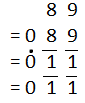Question 2: Divide by Dvajank method: 1234 ÷ 42

Solution: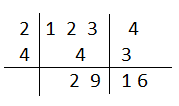Question 3: Evaluate the following product without multiplying directly.

96 × 97

Solution: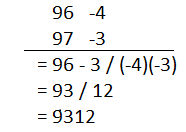Question 4: Change

$$\begin{array}{l}2\bar{4}\bar{3}\end{array}$$
into an ordinary number.

Solution: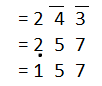Question 5: Divide by Paravartya Yajayet Method: 14885 ÷ 123

Solutions: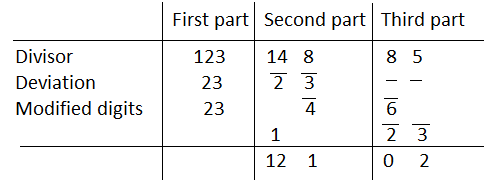Therefore, quotient = 121 and remainder = 02.

Question 6: Find the square of 103 by Duplex process or Dvanda yoga method.

Solution:

Square of 103:

= (1)2 / 2 × 1 × 0 / 2 × 1 × 3 + (0)2 / 2 × 0 × 3 / (3)2

= 1 / 0 / 6 / 0 / 9

= 10609

Question 7: Find the square by Duplex process or Dvanda yoga method for 29.

Solution:

Square of 29:

= (2)2 / 2 × 2 × 9 / (9)2

= 4 / 36 / 81

= 4 / 3 6 / 8 1

= 7 / 14 / 1

= 7 / 1 4 / 1

= 8 / 4 / 1

= 841

Question 8: Find the square of the number 96 by Duplex process or Dvanda yoga method.

Solution:

Square of 96:

= (9)2 / 2 × 9 × 6 / (6)2

= 81 / 108 / 36

= 81/ 10 8 / 3 6

= 91 / 11 / 6

= 91 / 1 1 / 6

= 92 / 1 / 6

= 9216

Question 9: Find the square of 97 by Duplex process or Dvanda yoga method.

Solution:

Square of 97:

= (9)2 / 2 × 9 × 7 / (7)2

= 81 / 126 / 49

= 81/ 12 6 / 4 9

= 93 / 10 / 9

= 93 / 1 0 / 9

= 94 / 0 / 9

= 9409

Question 10: Find the cube of 15 by Sutra Nikhilam base/sub-base method.

Solution:

Cube of 15:

= 15 + 2 × 5 / 3 × (5)2 / (5)3

= 15 + 10 / 3 × 25 / 125

= 25 / 75 / 125

= 25 / 7 5 / 12 5

= 32 / 17 / 5

= 32 / 1 7 / 5

= 33 / 7 / 5

= 3375

Question 11: Find the cube of 91 by Sutra Nikhilam base/sub-base method.

Solution:

Cube of 91:

= (9)3 / 3 × 1 × (9)2 / 3 × 9 × (1)2 / (1)3

= 729 / 3 × 81 / 27 / 1

= 729 / 243 / 27 / 1

= 729 / 24 3 / 2 7 / 1

= 753 / 5 / 7 / 1

= 753571

Question 12: Find the cube of the number 208 by Sutra Nikhilam base/sub-base method.

Solution:

Cube of 208:

= (2)2 [208 + 2 × (08)] / 3 × 2 × (08)2 / (08)3

= 4(208 + 16) / 6 × 64 / 512

= 4 × 224 / 384 / 512

= 896/ 3 84 / 5 12

= 899 / 89 / 12

= 8998912

Question 13: Find the cube of the number 32 by Sutra Nikhilam base/sub-base method.

Solution:

Cube of 32:

= (3)2 (32 + 2 × 2 ) / 3 × 3 × (2)2 / (2)3

= 9(32 + 4) / 9 × 4 / 8

= 9 × 36 / 36 / 8

= 324 / 3 6 / 8

= 327 / 6 / 8

= 32768